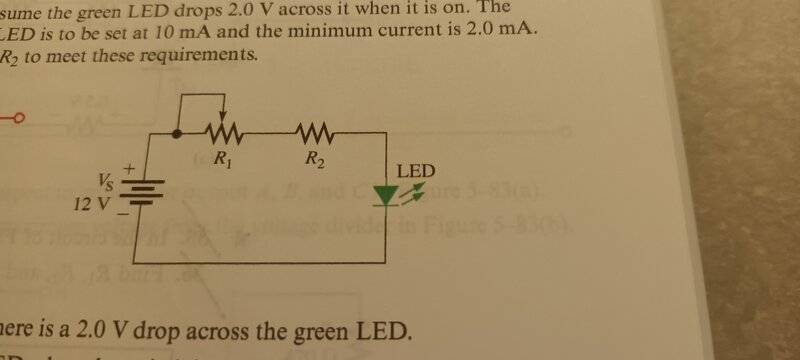# Understanding Voltage Drop in a Circuit

• Lay1
I will be careful in future and I will reply as you have explained. Thank you for your understanding and answer.f

#### Lay1

Homework Statement
Refer to the figure. Assume the green LED drops 2V across it when it is on. The maximum current in the LED is to be set at 10mA and the minimum current is 2mA. Choose values for R1 and R2 to meet these requirements.
Relevant Equations
V=IR
Here is the figure mentioned above.My thinking is that for maximum current, resistance must be minimum. Thus, R1 is not considered which means the voltage drop of R2 is 10V. So, R2 is 1kohm. For minimum, I=2mA, so R1+R2=5kohm, since R2 is 1kohm, R1 must be 4kohm. This is how I deduce. However, the answer shows that R1=5kohm while R2 is 1kohm. I do not understand why it is so. Could someone help me explain why? Thank you as always.

Last edited:
For minimum, I=2A, so R1+R2=5kohm, since R1 is 1kohm, R2 must be 4kohm. This is how I deduce.
Change the I=2A typo to I=2mA and I agree with you. I think the solution's answer for the minimum current case is incorrect.

Thus, R1 is not considered which means the voltage drop of R2 is 10V. So, R1 is 1kohm
R1 is not considered and R1 is 1kohm?

R1 is not considered and R1 is 1kohm?
Oh, good catch. Another typo!Yeah, we need the OP to add a reply with the R1/R2 stuff cleaned up.

Change the I=2A typo to I=2mA and I agree with you. I think the solution's answer for the minimum current case is incorrect.
Thank you for your guidance. And also thank you for your confirmation.

R1 is not considered and R1 is 1kohm?
I am really sorry for my careless mistake. And thank your for your correction.

Thank you for your guidance. And also thank you for your confirmation.
Wait, did you see where there are other typos as well? Please reply with the correct values for R1 and R2, where R2 is the fixed resistance to define the maximum current, and R1 is the variable resistance to define the minimum current when added to R2.

Oh, good catch. Another typo!Yeah, we need the OP to add a reply with the R1/R2 stuff cleaned up.

Wait, did you see where there are other typos as well? Please reply with the correct values for R1 and R2, where R2 is the fixed resistance to define the maximum current, and R1 is the variable resistance to define the minimum current when added to R2.
The textbook answer: R1=5kohms and R2=1kohm

••